### Home > A2C > Chapter 10 > Lesson 10.2.6 > Problem10-160

10-160.
1. In the diagram below, the point (x, y) is the same distance from (−3, 4) as it is from (2, −1). There are many possibilities for (x, y). Algebraically, what do they have in common? Homework Help ✎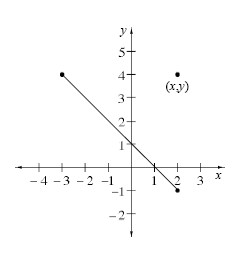1. What is the distance from (x, y) to (−3, 4)?

2. What is the distance from (x, y) to (2, −1)?

3. Write the equation that states that the two expressions in (a) and (b) are equal and simplify.

4. What is the specific name of the geometric object represented by your equation from part (c)?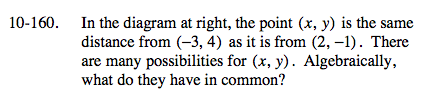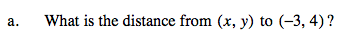Use the distance formula.

$\sqrt{(x + 3)^2+(y - 4)^2}$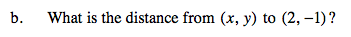See part (a).

$\sqrt{(x - 2)^2+(y + 1)^2}$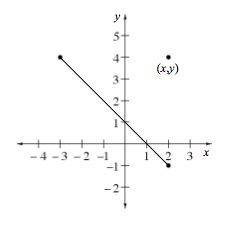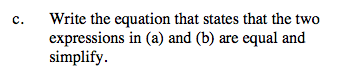$\sqrt{(x + 3)^2+(y - 4)^2} = \sqrt{(x - 2)^2+(y + 1)^2}$

(x + 3)2 + (y − 4)2 = (x − 2)2 + (y + 1)2

x2 + 6x + 9 + y2 − 8y + 16 = x2 − 4x + 4 + y2 + 2y + 1

10y = 10x + 20

y = x + 2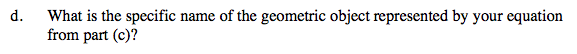Perpendicular bisector of the segment.